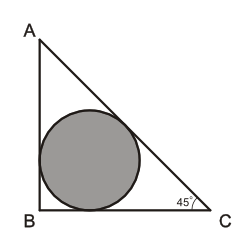We cover every section of the GMAT with in-depth lessons, 5000+ practice questions and realistic practice tests.

## Up to 90+ points GMAT score improvement guarantee

### The best guarantee you’ll find

Our Premium and Ultimate plans guarantee up to 90+ points score increase or your money back.

## Master each section of the test

### Comprehensive GMAT prep

We cover every section of the GMAT with in-depth lessons, 5000+ practice questions and realistic practice tests.

## Schedule-free studying

### Learn on the go

Study whenever and wherever you want with our iOS and Android mobile apps.

## The most effective way to study

### Personalized GMAT prep, just for you!The circle above is inscribed inside right triangle $$ABC$$. What is the radius of the circle if $$BC=8$$?
Incorrect. [[snippet]] Simplify the numerator first: remember, $$\sqrt{2}\approx 1.4$$. __Ballpark__ $$4\sqrt{2}$$ as $$4\cdot 1.4$$, which is slightly less than 6. Next, __Ballpark__ the denominator. Since >$$\sqrt{2} -1\approx 0.4$$ __Ballpark__ 0.4 as $$\frac{1}{2}$$, and estimate the fraction's value: >$$\displaystyle \frac{4\sqrt{2}}{\sqrt{2}-1} \approx \frac{6^-}{\frac{1}{2}^-} = 6 \div \frac{1}{2} = 6 \cdot \frac{2}{1} = 12$$. This is far greater than our __goal value__ of {color:purple}2.5{/color}. Eliminate and move on.
Incorrect. [[snippet]] Remember, $$\sqrt{2}\approx 1.4$$. __Ballpark__ $$4\sqrt{2}$$ as $$4\cdot 1.4$$, which is slightly less than 6. This is far greater than our __goal value__ of {color:purple}2.5{/color}. Eliminate and move on.
Correct. [[snippet]] Remember, $$\sqrt{2}\approx 1.4$$. __Ballpark__ $$4\sqrt{2}$$ as $$4\cdot 1.4$$, which is slightly less than 6. The result of subtracting this value from 8 should be slightly greater than 2, which is pretty close to our __goal value__ of {color:purple}2.5{/color}. This answer is a valid option. Move on and try to eliminate the other options. Since all of the other answer choices can be eliminated by either being too small or too big, this is the correct answer.
Incorrect. [[snippet]] Since $$\sqrt{2}+1$$ is greater than $$\sqrt{2}$$, this fraction is smaller than 1. This is far smaller than our __goal value__ of {color:purple}2.5{/color}. Eliminate it and move on.
Incorrect. [[snippet]] Since $$\sqrt{9}=3$$, $$\sqrt{8}$$ is slightly less than 3. Therefore, this fraction's numerator is smaller than its denominator, which means its value is less than 1. This is far smaller than our __goal value__. Eliminate it and move on.
$$\frac{\sqrt{8}}{4}$$
$$\frac{\sqrt{2}}{\sqrt{2}+1}$$
$$8-4\sqrt{2}$$
$$4\sqrt{2}$$
$$\frac{4\sqrt{2}}{\sqrt{2}-1}$$